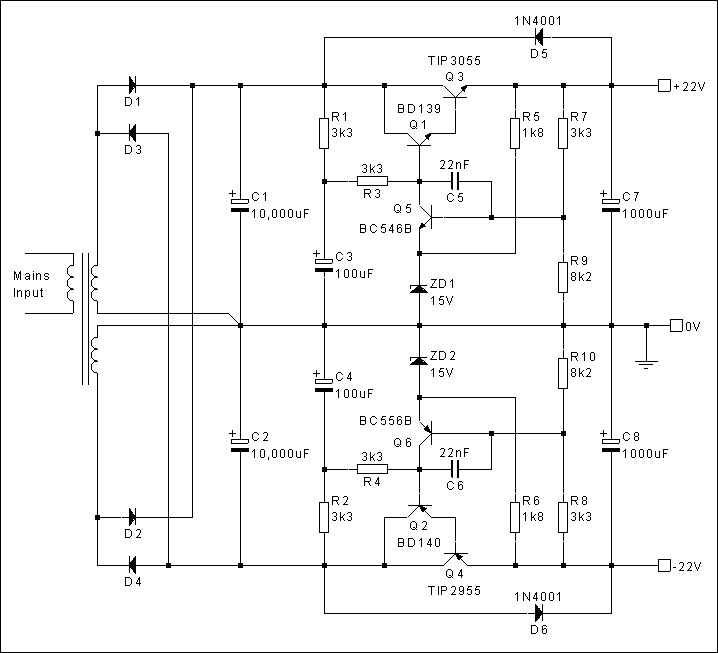The Class-A Amplifier Site

[ Back to Index ]

A Simple Voltage Regulator

The following diagram is provided for anyone who would like to include a voltage regulator but who does not want to use one of the ic versions (as in the updated, regulated supply for the 1996 design). This is a very basic regulator circuit, without any additional features such as foldback current limiting, and does not have the same performance as an ic regulator.Notes

The TIP2955/TIP3055 transistors should be satisfactory for a power supply feeding a single amplifier. If two amplifiers are to be fed from a single power supply, these transistors should be changed to higher power devices such as the MJ2955/2N3055. Adequate heat-sinking must be provided for whichever transistors are used.

R9 and R10 can be replaced with a 10k preset potentiometer (or a 5k potentiometer in series with a 5k6 fixed resistor) to provide adjustment of the output voltage.

As with the capacitance multiplier circuit, Q2 and Q4 can be changed to a complimentary feedback pair arrangement, if required, to allow the use 2N3055s as the pass device in both halves of the supply (see the capacitance multiplier page for details). If this done, R9 and R10 must be made variable to allow the supply rails to be set to equal (but opposite) voltages.

Zener diodes of a different voltage rating can be used for ZD1 and ZD2, but the value of R9 and R10 will need to be adjusted to maintain the +/-22V output.

For different output voltages or a different Zener diode voltage, the output voltage can be calculated from the following equations:

+VOUT = ((R7 + R9) / R9) * (VZ + 0.6)

-VOUT = ((R8 + R10) / R10) * (VZ + 0.6)

where +VOUT and -VOUT are the required supply rail voltages and VZ is the Zener voltage.

[ Back to Index ]

HISTORY: Page created 13/05/2001

16/05/2001 Diagram amended to correct polarity of D6, Resistor numbering corrected in voltage equation

17/05/2001 Minor text changes. Second voltage equation added